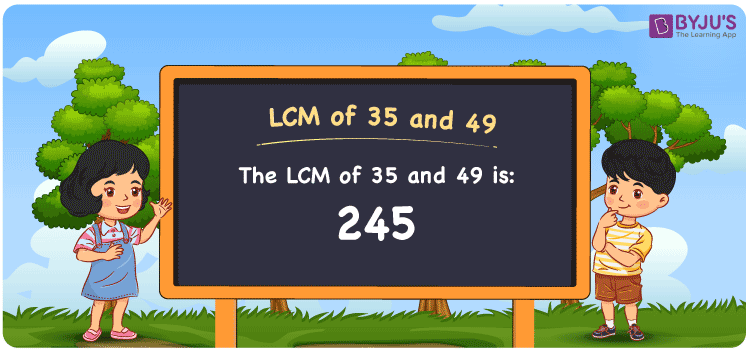# LCM of 35 and 49

LCM of 35 and 49 is 245. LCM, also known as Least Common Multiple or Lowest Common Multiple, is the smallest numeral that is divisible by the given numbers. In the given set of numbers 35 and 49, 245 is the least number that is divisible by both 35 and 49. Therefore the LCM or LCD also known as the Least Common divisor for 35 and 49 is 245. You can refer to LCM for more information.

## What is LCM of 35 and 49

The Least Common Multiple or Lowest Common Multiple of 35 and 49 is 245.## How to Find LCM of 35 and 49?

LCM of 35 and 49 can be determined using three methods:

• Prime Factorisation
• Division method
• Listing the multiples

### LCM of 35 and 49 Using Prime Factorisation Method

In the Prime Factorisation method, the numbers 35 and 49 can be expressed as;

35 = 5 × 7

49 = 7 × 7

7 is the common factor for both 35 and 49, along with other factors of 35 and 49 are multiplied to get the LCM.

LCM (35, 49) = 5 × 7 × 7 = 245

### LCM of 35 and 49 Using Division Method

In the Division Method, the numbers 35 and 49 are divided by common and uncommon factors. The product of these common and uncommon factors forms the LCM.

 5 35 49 7 7 49 7 1 7 × 1 1

LCM (35, 49) = 5 × 7 × 7= 245

### LCM of 35 and 49 Using Listing the Multiples

By listing all the multiples of 35 and 49, we can identify the LCM. Below is the list of multiples for 35 and 49

 Multiples of 35 Multiples of 49 35 49 70 98 105 147 140 196 175 245 210 294 245 343 280 392

LCM (35, 49) = 245

## Video Lesson on Applications of LCM## Solved Examples

What is the smallest number that is divisible by both 35 and 49?

Answer: 245 is the smallest number that is divisible by both 35 and 49.

Is 245 a multiple of 35 and 49?

Answer: Yes. 245 is a multiple of 35 and 49.

## Frequently Asked Questions on LCM of 35 and 49

### What is the LCM of 35 and 49?

The LCM of 35 and 49 is 245.

### Is the LCM of 35 and 49 the same as the Highest Common Factor of 35 and 49?

No. LCM of 35 and 49 is 245 and Highest Common Factor of 35 and 49 is 7.

### What are the common prime factors of 35 and 49?

7 is the common prime factor of 35 and 49.

### What are the first 3 common multiples of 35 and 49?

The first three common multiples of 35 and 49 are 245, 490, and 735.

### Is the LCM of 7 and 35 the same as the LCM of 7 and 49?

No. LCM of 7 and 35 is 35, whereas the LCM of 7 and 49 is 49. They are not the same.